## Example Questions

### Example Question #1 : Proportion / Ratio / Rate

A rectangular container holds a liquid. The dimensions of the container are 5 cm by 5 cm by 4 cm. If the container is half full, how much liquid is in the container (1 cm= 1mL)?

25 mL

100 mL

20 mL

150 mL

50 mL

50 mL

Explanation:

The total volume is 5 * 5 * 4 = 100 cm. Half of this is 50 cmwhich is 50 mL.

### Example Question #2 : Proportion / Ratio / Rate

A dog eatstreats indays. At this rate, how many treats does the dog eat in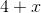days?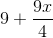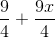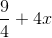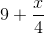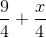Explanation:

This is a rate problem. We need to first find out how many treats a day the dog eats. Then to find the number of treats the dog eats indays, we multiply the number of days by the number of treats a day the dog eats.

From the given information, we know that the dog eats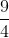treats a day.

Then we multiply that number by the number of days.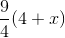Now simplify.### Example Question #3 : Proportion / Ratio / Rate

One serving of party drink is comprised of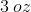of syrup,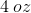of water, andof apple juice.  If a large bowl of the drink contains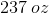of apple juice, how much of the total drink is in the bowl?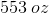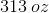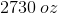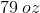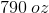Explanation:

The total drink is made up of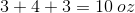. Therefore, for a problem like this, you can set up a ratio: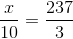First, simplify the right side of the equation: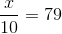Next, solve for: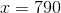.  Therefore, the total amount of drink is.

### Example Question #4 : Proportion / Ratio / Rate

There is a coffee drink made ofof coffee,of milk,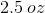of cream, and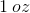of flavoring. If you have an unlimited amount of flavoring and milk but only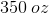of cream and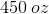of coffee, how many ounces of drink can you make? (Presume that you cannot make partial servings.)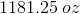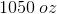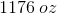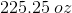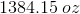Explanation:

To begin with, you need to compute what is going to be your limiting factor. For the cream, you can make: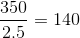servings.

For the coffee, you can make: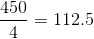servings.

Therefore, this second value is your total number of servings. (You must choose the minimum, for it will be what limits your beverage-making.)

So, you know that each drink is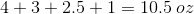.  If you can make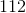servings (remember, no partial servings!), you can make a total of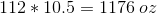.

### Example Question #5 : Proportion / Ratio / Rate

A drink is made up of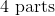orange juice to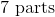carbonated water.  If a bowl of this drink contains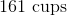of carbonated water, how many total cups of the drink are there in the bowl?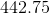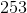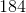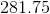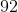Explanation:

To start this, you can set up a proportion as follows: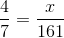, whereis the number of cups of orange juice.

Now, solving for, you get: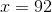Be careful, though! This means that the total solution is actually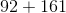or.

### Example Question #6 : Proportion / Ratio / Rate

A solution has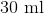of solution X and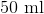of solution Y.  If you wanted a solution containing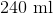of solution Y, how much total solution would you need?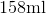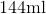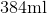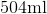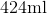Explanation:

To start, notice that the ratio of solution X to solution Y is:Based on the quesiton, you know that you are looking for a certain amount of solution X based on a given amount of solution Y. Thus, for your data, you know: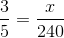Solving for X, you get: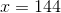This is the total amount of solution X that you will need to keep the ratios correct. Do not forget that you need to have a total solution amount, thus add this amount of solution X to solution Y's amount, thus giving you: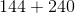or